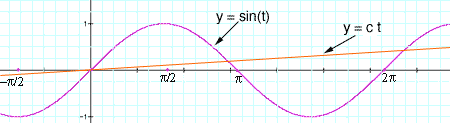Quandaries and Queries What is an integer value for k so that sin(kx) = x has exactly 2005 solutions? How does one arrive at the answer? -David Mathematics Teacher Hi David, I don't believe there is such an integer k. To show you my argument I want to make a change of variables. Let t = kx then the equation is sin(t) = 1/k t I now want to write c= 1/k so I don't have to type fractions. The problem then is to find c so that sin(t) = ct where c is the reciprocal of an integer. y = ct is a straight line through the origin with slope c so you want to find c so that the sine curve and the line intersect exactly 2005 times.From the diagram you can see that the line cuts the curve 3 times for -2< t < 2the line cuts the curve 3 + 4 = 7 times for -4< t < 4the line cuts the curve 3 + 24 = 11 times for -6< t < 6the line cuts the curve 3 + 34 = 15 times for -8< t < 8. . the line cuts the curve 3 + 5004 = 2003 times for -1002< t < 1002Hence the line must cut the curve exactly twice more, once to the right of the origin and once to the left. Thus, to the right of the origin in the next loop of the sine function (1002< t < 1004) the line must cut the curve exactly once. Hence the line is tangent to the curve at this point. This means that there is exactly one number c so that the line intersects the curve at exactly 2005 points. My difficulty is that I see no reason why c should be the reciprocal of an integer! I tried to solve for c numerically but I was successful. Harley